# How many grams of dry NH4Cl need to be added to 2.50 L of a 0.500 M solution of ammonia, NH3, to prepare a buffer soluti...

How many grams of dry NH4Cl need to be added to 2.50 L of a 0.500 M solution of ammonia, NH3, to prepare a buffer solution that has a pH of 8.74? Kb for ammonia is 1.8*10^-5.

Concepts and reason

Buffer:

A buffer is a chemical solution that contains a weak acid/base mixed with its conjugate base/acid (as in the form of salts). It can resist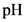change when a small amount of a strong acid/base is added to it. The acidic buffer solution is made up of less than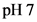and the alkali buffer solution is made up of more than.

Fundamentals

Henderson-Hasselbalch equation is a mathematical expression which can be used to calculate theof buffer solutions.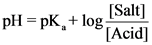Concentration of acid and base can be calculated as follows:Write the Henderson-Hasselbalch equation of basic buffer calculation.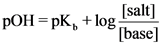Concentration of acid and base can be calculated as follows:The reaction for given buffer solution can be written as follows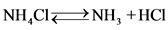Given data: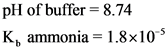So,Then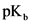of given solution can be calculated as followsHenderson-Hasselbalch was used for calculating the mass of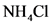as given below, the values of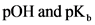were substituted in Henderson-Hasselbalch equation.ThenFinally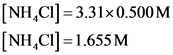The mole of ammonium chloride was calculated as follows: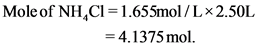The mass of ammonium chloride is calculated as follows: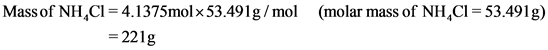Ans:

The mass of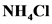is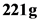.

##### Add Answer of: How many grams of dry NH4Cl need to be added to 2.50 L of a 0.500 M solution of ammonia, NH3, to prepare a buffer soluti...
Similar Homework Help Questions
• ### How many grams of dry NH4Cl need to be added to 1.50 L of a 0.500 M solution of ammonia, NH3,to prepare a buffer solutio...

How many grams of dry NH4Cl need to be added to 1.50 L of a 0.500 M solution of ammonia, NH3,to prepare a buffer solution that has a pH of 8.79? Kb for ammonia is 1.8*10^-5.

• ### How many grams of dry NH4Cl need to be added to 2.50 L of a 0.400 M solution of ammonia, NH3, to prepare a buffer solu...

How many grams of dry NH4Cl need to be added to 2.50 L of a 0.400 M solution of ammonia, NH3, to prepare a buffer solution that has a pH of 8.67? Kb for ammonia is 1.8×10−5.

• ### How many grams of dry NH4Cl need to be added to 2.10 L of a 0.500 M solution of ammonia, NH3, to prepare a buffer solu...

How many grams of dry NH4Cl need to be added to 2.10 L of a 0.500 M solution of ammonia, NH3, to prepare a buffer solution that has a pH of 8.76? Kb for ammonia is 1.8×10−5. Express your answer with the appropriate units.

• ### How many grams of dry NH4Cl need to be added to 1.70 L of a 0.100 M solution of ammonia, NH3, to prepare a buffer solu...

How many grams of dry NH4Cl need to be added to 1.70 L of a 0.100 M solution of ammonia, NH3, to prepare a buffer solution that has a pH of 8.62? Kb for ammonia is 1.8×10−5.

• ### How many grams of dry NH4Cl need to be added to 2.30 L of a 0.100 M solution of ammonia,NH3, to prepare a buffer soluti...

How many grams of dry NH4Cl need to be added to 2.30 L of a 0.100 M solution of ammonia,NH3, to prepare a buffer solution that has a pH of 9.00? Kb for ammonia is 1.8x 10^-5.

• ### How many grams of dry NH4Cl need to be added to 2.40 L of a 0.400 M solution of ammonia, NH3, to prepare a buffer s...

How many grams of dry NH4Cl need to be added to 2.40 L of a 0.400 M solution of ammonia, NH3, to prepare a buffer solution that has a pH of 8.78? Kb for ammonia is 1.8 x 10^-5.

• ### How many grams of dry NH4Cl need to be added to 2.00 L of a 0.700...

How many grams of dry NH4Cl need to be added to 2.00 L of a 0.700 M solution of ammonia, NH3, to prepare a buffer solution that has a pH of 9.00? Kb for ammonia is 1.8×10−5. Express your answer with the appropriate units.

• ### Part B How many grams of dry NH4Cl need to be added to 1.90 L of...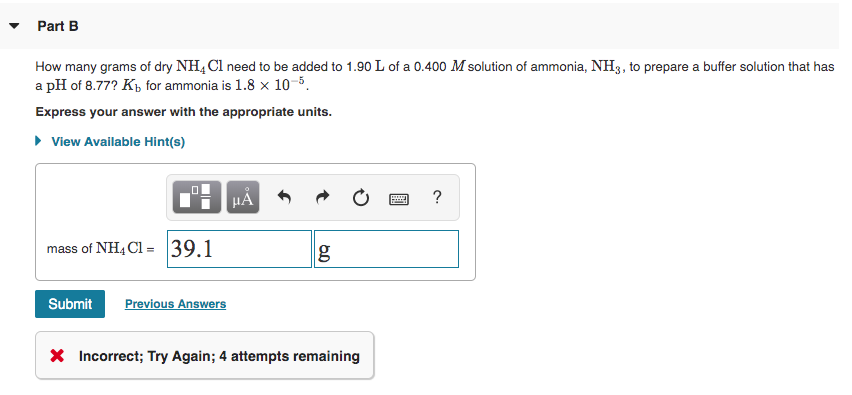Part B How many grams of dry NH4Cl need to be added to 1.90 L of a 0.400 M solution of ammonia, NH3, to prepare a buffer solution that has a pH of 8.77? Kb for ammonia is 1.8 x 10-5 Express your answer with the appropriate units. View Available Hint(s) CHÅN O a? mass of NH4C1 = 39.1 g Submit Previous Answers X Incorrect; Try Again; 4 attempts remaining

• ### Part A: How many grams of dry NH4Cl need to be added to 2.40 L of...

Part A: How many grams of dry NH4Cl need to be added to 2.40 L of a 0.500 M solution of ammonia, NH3, to prepare a buffer solution that has a pH of 8.71? Kb for ammonia is 1.8×10^−5. Part B: A 75.0-mL volume of 0.200 M NH3 (Kb=1.8×10−5) is titrated with 0.500 M HNO3. Calculate the pH after the addition of 17.0 mL of HNO3. Part C: Barium sulfate, BaSO4, is used in medical imaging of the gastrointestinal tract...

• ### Buffer Problem

How many grams of dry NH4Cl need to be added to 2.50 L of a 0.700 M solution of ammonia, NH3, to prepare a buffer solution that has a pH of 8.99? Kb for ammonia is1.8x10^-5

Need Online Homework Help?